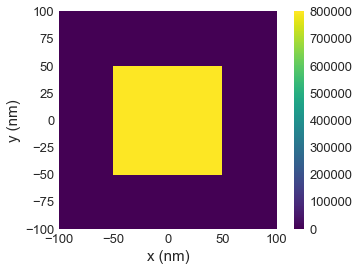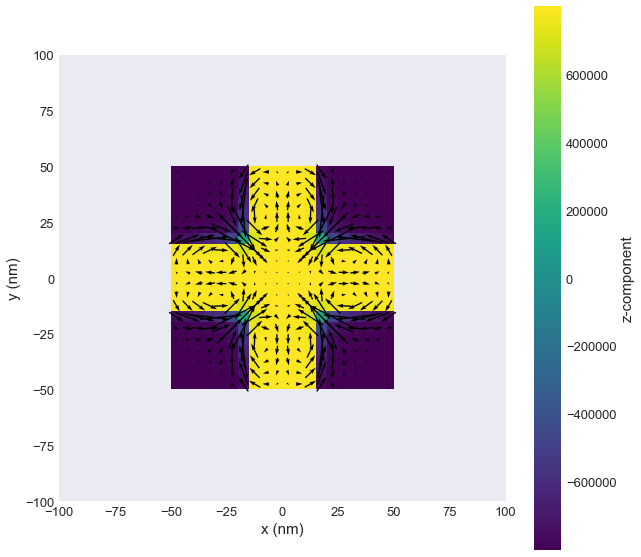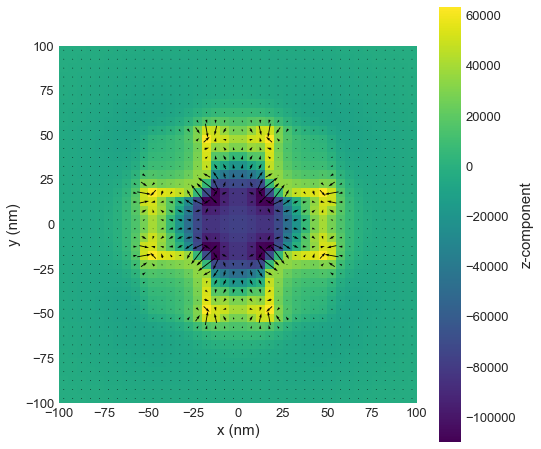# Tutorial 12: Calculating a stray field using an airbox method¶

Interactive online tutorial:In order to calculate the stray field outside the sample, we have to define an "airbox" which is going to contain our sample. In this example we define a box with 100 nm edgle length as a mesh which then contains a magnetic sample which is a cube with 50 nm dimensions. We achieve this by implementing a Python fuction for defining the Ms (norm_fun). Outside our sample the value of saturation magnetisation is zero.

In :
import discretisedfield as df
import micromagneticmodel as mm
import oommfc as oc
%matplotlib inline

region = df.Region(p1=(-100e-9, -100e-9, -100e-9), p2=(100e-9, 100e-9, 100e-9))
mesh = df.Mesh(region=region, cell=(5e-9, 5e-9, 5e-9))

def norm_fun(pos):
x, y, z = pos
if -50e-9 <= x <= 50e-9 and -50e-9 <= y <= 50e-9 and -50e-9 <= z <= 50e-9:
return 8e5
else:
return 0

system = mm.System(name='airbox_method')
system.energy = mm.Exchange(A=1e-12) + mm.Demag()
system.dynamics = mm.Precession(gamma0=mm.consts.gamma0) + mm.Damping(alpha=1)
system.m = df.Field(mesh, dim=3, value=(0, 0, 1), norm=norm_fun)


We can now plot the norm to confirm our definition.

In :
system.m.norm.plane('z').mpl()In the next step, we can relax the system and show its magnetisation.

In :
md = oc.MinDriver()
md.drive(system)

system.m.plane('z').mpl(figsize=(10, 10))

Running OOMMF (ExeOOMMFRunner) [2020/06/12 00:55]... (7.2 s)Stray field can now be calculated as an effective field for the demagnetisation energy.

In :
stray_field = oc.compute(system.energy.demag.effective_field, system)

Running OOMMF (ExeOOMMFRunner) [2020/06/12 00:55]... (2.2 s)


stray_field is a df.Field and all operations characteristic to vector fields can be performed.

In :
stray_field.plane('z').mpl(figsize=(8, 8), vector_scale=1e6)